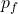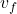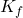Question

A space vehicle of mass m has a speed v. At some instant, it separates into two pieces, each of mass 0.5m. One of the pieces is at rest just after the separation. How much work was done by the internal forces that caused the separation

1.W = ½ m v²

Explanation:

In this exercise we must solve it in parts, in a first part we use the conservation of the moment to find the speed after the separation

We define the system formed by the two parts of the rocket, therefore the forces during internal separation and the moment are conserved

initial instant. before separation

p₀ = m v

final attempt. after separation= m /2  0 + m /2 v_{f}

p₀ = p_{f}

m v = m /2v_{f}= 2 v

this is the speed of the second part of the ship

now we can use the relation of work and energy, which establishes that the work is initial to the variation of the kinetic energy of the body

initial energy

K₀ = ½ m v²

final energy= ½ m/2  0 + ½ m/2 v_{f}²

K_{f} = ¼ m (2v)²

K_{f} = m v²

the expression for work is

W = ΔK = K_{f} – K₀

W = m v² – ½ m v²

W = ½ m v²